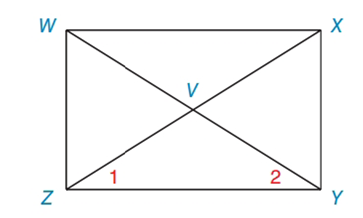Chapter 4.3, Problem 24E### Elementary Geometry for College St...

6th Edition
Daniel C. Alexander + 1 other
ISBN: 9781285195698

#### Solutions

Chapter
Section### Elementary Geometry for College St...

6th Edition
Daniel C. Alexander + 1 other
ISBN: 9781285195698
Textbook Problem
1 views

# In Exercises 23 and 24, supply the missing statements and reasons. Given: ▭ W X Y Z  with diagonals  W Y ¯  and  X Z ¯ Prove: ∠ 1 ≅ ∠ 2PROOF Statements Reasons 1. ? 1. Given 2. ? 2. The diagonals of a rectangle are ≅ 3. W Z ¯ ≅ X Y ¯ 3. The opposite sides of a rectangle are ≅ 4. Z Y ¯ ≅ Z Y ¯ 4. ? 5. △ X Z Y ≅ △ W Y Z 5. ? 6. ? 6. ?

To determine

To supply:

The missing statements and reasons and prove 12.

Explanation

SSS Rule for triangle congruency:

Calculation:

Consider the rectangle.

 PROOF Statements Reasons 1. WXYZ is a rectangle with diagonals of WY and XZ. 1. Given 2. WY is congruent to XZ.WY¯≅ZX¯ 2. The diagonals of a rectangle are ≅ 3

### Still sussing out bartleby?

Check out a sample textbook solution.

See a sample solution

#### The Solution to Your Study Problems

Bartleby provides explanations to thousands of textbook problems written by our experts, many with advanced degrees!

Get Started

#### Find more solutions based on key concepts